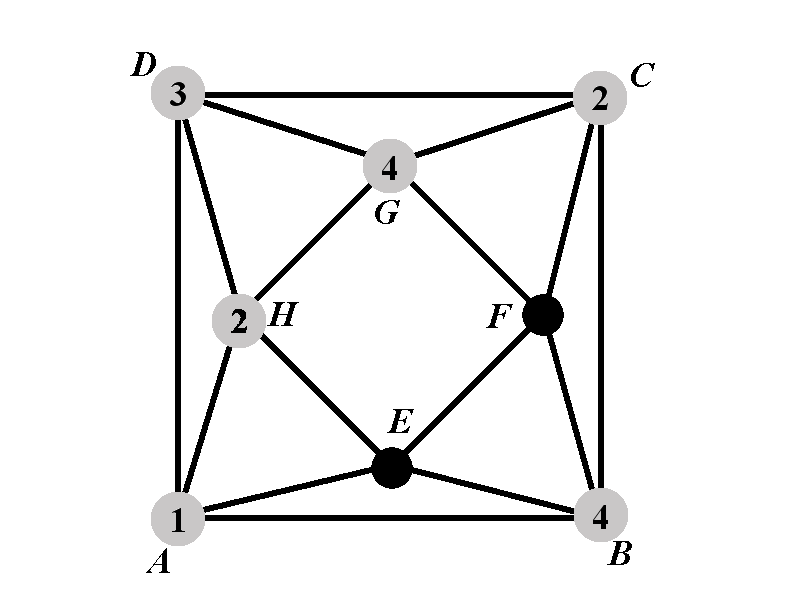Back to: Chromatic Polynomial Case $$2.2.1^{\circ}$$
##### Case 2.2.1.2: Calculation of $$P_{2.2.1.2^{\circ}}(k)$$Observe that the vertex $$E$$ cannot have any of the colors $$1$$, $$2$$, and $$4$$. There are $$k-3$$ ways to assign a color to $$E$$. The color assigned to $$F$$ will not be $$2$$ nor $$4$$. Thus, each assignment of the color to the vertex $$E$$ leaves exactly $$k-3$$ possible colors for $$F$$. The vertex $$F$$ cannot have color $$2$$, nor color $$4$$, nor the color assigned to $$E$$. Therefore $P_{2.2.1.2^{\circ}}(k)=(k-3)^2.$